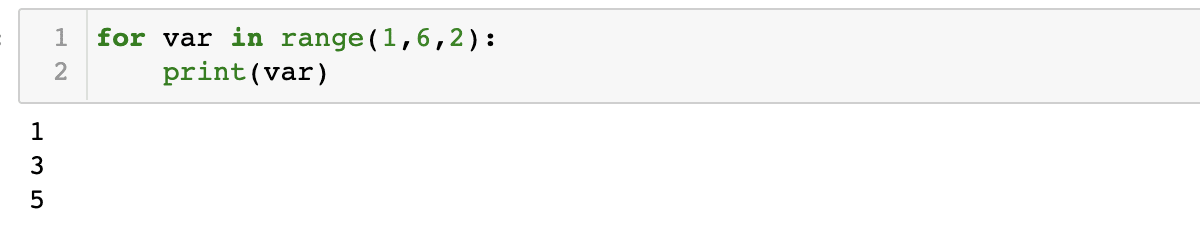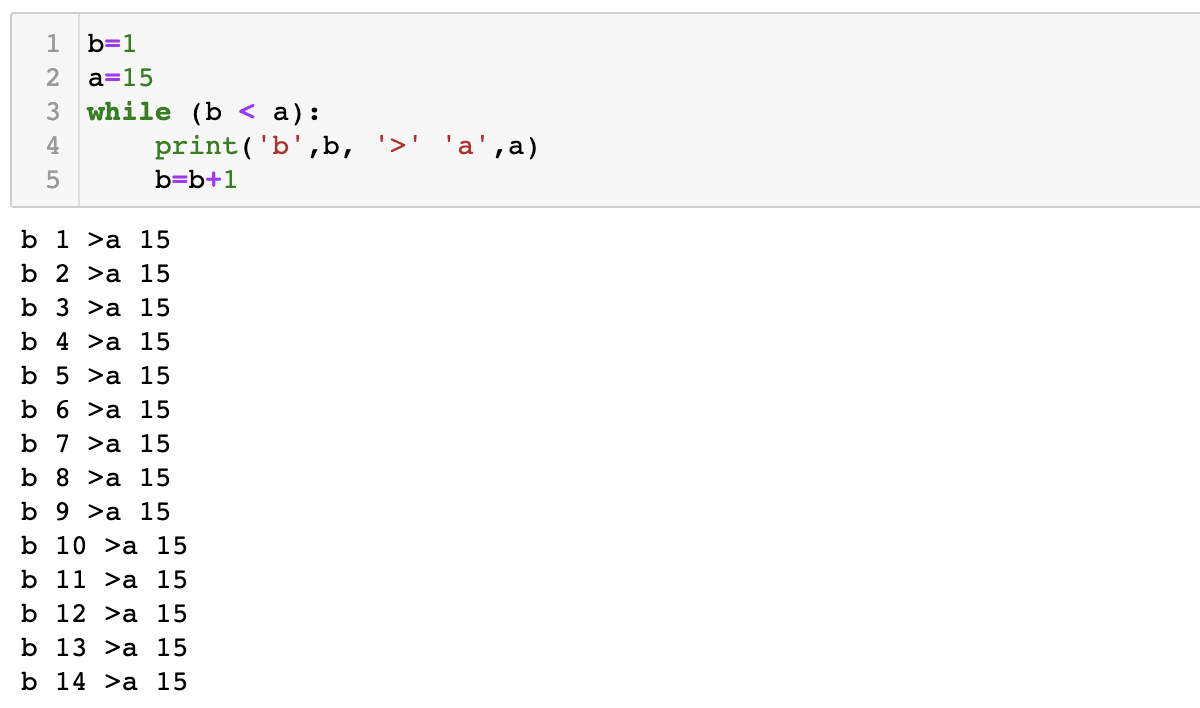Skip to content

# Conditionals

Conditionals are statements that we include in our code to allow for decision making. Depending on the use case, we ask our code to return different outcomes. Common examples of conditionals include, ‘if’, ‘for’, and ‘while’.

## If Conditional

Example: We will be using an if statement to identify the larger number of two. Based on the result, we will carry out different print statements.

We are defining our variables a=5 and b=6. We then check the condition: is a greater than b? If this condition is true, then the statements indented from this if statement will be executed. If it is false, we jump to the second if condition, ‘elif'(else if). The same process of checking occurs for this statement. Finally, if none of the conditions statements are true, the code executes the final ‘else’ statement.

```a=5
b=6
if (a > b):
print ("a is greater than b")
print ("blocks are defined by indentation")
elif (a < b):
print ("a is less than b")
else:
print ("a is equal to b")```Remember to indent the statements after each conditional!

Additionally, conditionals can be used to perform a function multiple i.e. on a loop. Looping statements can be very useful in certain decision making processes as well. To understand the usage of each, let’s look at a few examples.

## For Conditionals

For functions are used to specify certain actions when the given condition is true. In this example, the range function is used. Range can take the following syntax: `range(start, finish, increment)`.

Example: We specify that the range must start at one and end at 6, with increments of 2. ‘var’ can be any variable. The stop point of the range function must be given. By default the starting position is taken as 0 and increments of 1 are assumed if not specified.  If we print this we can observe the following result:

```for var in range(1,6,2):
print(var)```For the given range, perform this action.

## While Conditionals

Executes statements while the expression is true.

Example: We are instructing the code to print b while b is less than a. We then increment b by 1. When b is the same as a, the iteration will stop.

```b=1
a=15
while (b < a):
print('b',b, '>' 'a',a)
b=b+1```## Some other conditional statements

continue: Skips the rest of the body of the loop for the current iteration. In the next iterations the loop will continue normally.

break: Ends the execution of the current loop.

else: for and while loops can both have else clauses, which are executed after the loop terminates normally by falsifying the conditional, but else clauses are not executed when a loop terminates via a break statement.

foreach: for x in array: statement
Loops over the array given by array. On each iteration, the value of the current element is assigned to x and the internal array pointer is advanced by one.